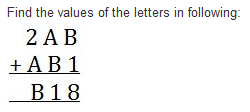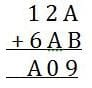Test: Playing With Numbers- 2

# Test: Playing With Numbers- 2

Test Description

## 20 Questions MCQ Test Mathematics (Maths) Class 8 | Test: Playing With Numbers- 2

Test: Playing With Numbers- 2 for Class 8 2022 is part of Mathematics (Maths) Class 8 preparation. The Test: Playing With Numbers- 2 questions and answers have been prepared according to the Class 8 exam syllabus.The Test: Playing With Numbers- 2 MCQs are made for Class 8 2022 Exam. Find important definitions, questions, notes, meanings, examples, exercises, MCQs and online tests for Test: Playing With Numbers- 2 below.
Solutions of Test: Playing With Numbers- 2 questions in English are available as part of our Mathematics (Maths) Class 8 for Class 8 & Test: Playing With Numbers- 2 solutions in Hindi for Mathematics (Maths) Class 8 course. Download more important topics, notes, lectures and mock test series for Class 8 Exam by signing up for free. Attempt Test: Playing With Numbers- 2 | 20 questions in 20 minutes | Mock test for Class 8 preparation | Free important questions MCQ to study Mathematics (Maths) Class 8 for Class 8 Exam | Download free PDF with solutions
 1 Crore+ students have signed up on EduRev. Have you?
Test: Playing With Numbers- 2 - Question 1

### If the three digit number 24x is divisible by ‘9’, what is the value of ‘x’?

Detailed Solution for Test: Playing With Numbers- 2 - Question 1

For a number to be divisible by 9, the sum of its digits must be divisible by 9.

Here, 2 + 4 = 6. Next multiple of 9 is 9.

Therefore, we will add 9 -  6 = 3. So,  the value of x = 3.

Test: Playing With Numbers- 2 - Question 2

### Which of the numbers is divisible by 10?

Test: Playing With Numbers- 2 - Question 3

### By which of the following number 9042 is not divisible? 2, 3, 6, and 9

Detailed Solution for Test: Playing With Numbers- 2 - Question 3 Correct answer is'C' because the sum of 9042 is not a multiple of 9.
Test: Playing With Numbers- 2 - Question 4

Identify the missing digit in the number 234,4_6, if the number is divisible by 4.

Test: Playing With Numbers- 2 - Question 5

Determine if 48 is divisible by 2, 3 and 5.

Detailed Solution for Test: Playing With Numbers- 2 - Question 5 Yes, the B option is right because if you divide 48 by 2 and 3 it is easy to divide and reminder is 0 but if you divide 48 by 5 it is not divide. Thats why 48 is divisible by 2 and 3.
Test: Playing With Numbers- 2 - Question 6

By which of these numbers is the number 3116365 divisible?

Detailed Solution for Test: Playing With Numbers- 2 - Question 6

The last digit is 5, the number is divisible by 5.

Since

311636 − 10 = 311626 ÷ 7 = 44568,

the number is divisible by 7 also.

Test: Playing With Numbers- 2 - Question 7

Write in generalised form: 25

Test: Playing With Numbers- 2 - Question 8

Which of the following statements is false?

Detailed Solution for Test: Playing With Numbers- 2 - Question 8

A number divisible by 3 and 4 is divisible by 12.

Test: Playing With Numbers- 2 - Question 9

If the division N ÷ 5 leaves a remainder of 0, what might be the one’s digit of N?

Detailed Solution for Test: Playing With Numbers- 2 - Question 9

Solution :- If a number is divisible by 5 then it's unit digit must be 0 or 5. so if we need the remainder of 0 when divided by 5 then the numbers unit digit must be 0 or 5.

Test: Playing With Numbers- 2 - Question 10

Write in the usual form: 10×5 + 6

Detailed Solution for Test: Playing With Numbers- 2 - Question 10

10 ×5 = 50
6 = 6
⇒ 50 + 6 = 56

Test: Playing With Numbers- 2 - Question 11Detailed Solution for Test: Playing With Numbers- 2 - Question 11

B+1=8
B=7, A+7=-1 .So A=4 and B=7

Test: Playing With Numbers- 2 - Question 12

The number 21436587 is divisible by _____.

Test: Playing With Numbers- 2 - Question 13

Write in generalised form: 73

Test: Playing With Numbers- 2 - Question 14

Write in the usual form: 100 × 7 + 10 × 1 + 8

Test: Playing With Numbers- 2 - Question 15

Which of these numbers is divisible by 6?

Detailed Solution for Test: Playing With Numbers- 2 - Question 15

Divisible by 2 and 3 both.

Test: Playing With Numbers- 2 - Question 16

If the division N ÷ 2 leaves no remainder (i.e., zero remainder), what might be the one’s digit of N?

Detailed Solution for Test: Playing With Numbers- 2 - Question 16

Since every multiple of 2 leaves no remainder when divided by 2.Therefore N can be ,0,2,4,6 or 8

Test: Playing With Numbers- 2 - Question 17

Find the value of the letters in following:Test: Playing With Numbers- 2 - Question 18

Write in generalised form: 85

Test: Playing With Numbers- 2 - Question 19

Write in the usual form: 100 × a + 10 × c + b

Detailed Solution for Test: Playing With Numbers- 2 - Question 19

We can write acb=100*a+10*c+b which say that a is at hundred’s place, c is at ten’s place and b is at one’s place.

Test: Playing With Numbers- 2 - Question 20

If the division N ÷ 5 leaves a remainder of 4, what might be the one’s digit of N?

Detailed Solution for Test: Playing With Numbers- 2 - Question 20

Since every multiple of 5 ends with either 5 or 0. Therefore, the unit digit of N must be either 0+4=4 or 5+4=9. Hence the answer is either 4 or 9.

## Mathematics (Maths) Class 8

187 videos|321 docs|48 tests
 Use Code STAYHOME200 and get INR 200 additional OFF Use Coupon Code
Information about Test: Playing With Numbers- 2 Page
In this test you can find the Exam questions for Test: Playing With Numbers- 2 solved & explained in the simplest way possible. Besides giving Questions and answers for Test: Playing With Numbers- 2, EduRev gives you an ample number of Online tests for practice

## Mathematics (Maths) Class 8

187 videos|321 docs|48 tests